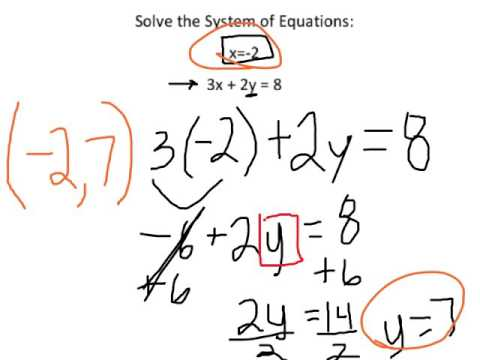### 12.9 Systems of Equations (Substitution)# 12.9 Systems of Equations (Substitution)

## 22 Video Interactions

1. 00:00:16

What are the THREE ways to solve a system of equations?

• Graphing

• Substitution

• Elimination

• Multiply the equations

• As a friend to solve it for you

• Divide the equations to eliminate a variable

2. 00:00:19

What method should we use to solve this problem?

• Elimination

• Substitution

3. 00:00:27

Which variable will we substitute for?

• y

• x

4. 00:00:32

What will we substitute for x?

• -2

5. 00:00:35

We are going to substitute for  to find

6. 00:00:42

Re-write the second equation substituting for x

____________________________

7. 00:00:54

What is our next step?

____________________________

8. 00:01:05

Which variable do I need to solve for?

• x

• y

9. 00:01:15

What do I do next?

• Subtract 8 from both sides

• Add 6 to both sides

10. 00:01:43

How do I need to write my solution?

• x=    and y=

• As coordinates

• As a fraction

• In y=mx+b form

11. 00:01:46

( , )

• -2

12. 00:02:28

What are TWO ways you could solve this problem?

____________________________

13. 00:02:52

What is the initial value for company A?

• 5

• 30

• 0

14. 00:03:01

What is my unit rate (m)?

• 5

• 30

• 0

15. 00:03:10

What is the initial value for company b?

• 0

• 10

• 5

• 30

16. 00:03:17

write your equation for company B

____________________________

17. 00:03:25

What does x represent in the equation?

• Number of hours you use your phone

• Number of gigs of data used

• Sign-up fee

• Cell phone company A and cell phone company B

18. 00:03:52

If we are trying to find the number of gigs where the two companies are the same, which variable are we solving for?

• y

• x

19. 00:04:29

I can substitute this 5x+3...

• for x

• for y

• nowhere

20. 00:04:45

Substitute 5x+30 for y

____________________________

21. 00:05:12

What do I need to do now?

• Subtract 10x from both sides

• Subtract 30 from both sides

• Subtract 5x from both sides

• Divide both sides by 10

22. 00:05:43

How many gigs of data will the two companies cost the same?

• 6 gigs

Click here to preview, use, or make a copy of this interactive video lesson plan (bulb).